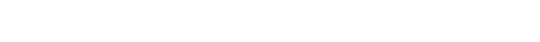For the equilibria between an ionic solid and its dissolved ions, the equilibrium constant is called the solubility product Ksp.Ksp gives us information about the solubility of the compound. The lower Ksp is, the less soluble a compound is.

Eg: CuCl2 Ksp=1.7×10-7 at 25°C        NaCl Ksp= 3.8×101 at 25°C      CuS Ksp=6.0×10-37 at 25°C

Solubility: The concentration of a dissolved solid in g/100mL. If stated in M is called molar solubility.

Calculating Ksp from solubilityCalculate solubility using KspPredicting Precipitation:The reaction quotient can tell us whether a compound will form a precipitate or not by comparing it to Ksp.

• If Q = K the solution is saturated and there should be no precipitate formed
• If Q < K, the solution is unsaturated and there should be no precipitate formed
• If Q > K, the solution is supersaturated and the equilibrium will shift to the left to reduce the amount of products, creating a precipitate

Ex: if 100 mL of 0.100 M calcium chloride are mixed with 100 mL of 0.0400 M sodium sulfate at 20°C, will a precipitate form? Hint, the Ksp of calcium sulfate is 3.6×10-5 at 20°C.

Since Q > K, a precipitate will form.

Cite this article as: William Anderson (Schoolworkhelper Editorial Team), "Solubility Product Constant," in SchoolWorkHelper, 2019, https://schoolworkhelper.net/solubility-product-constant/.